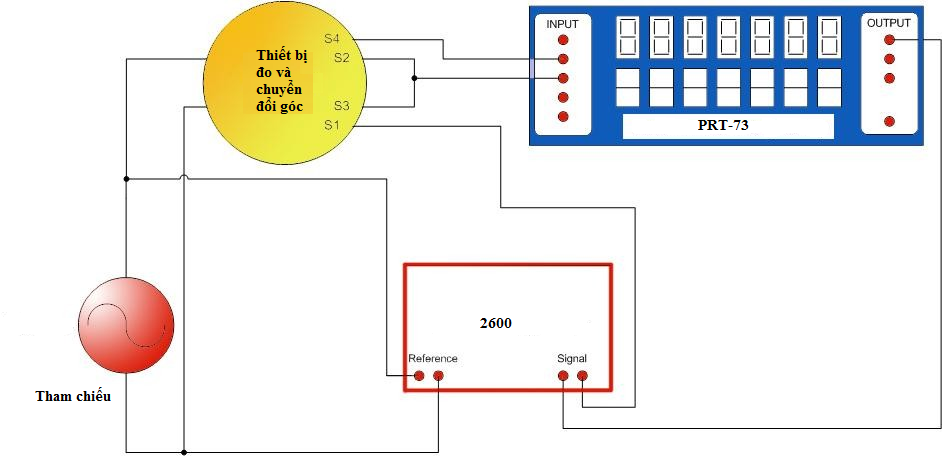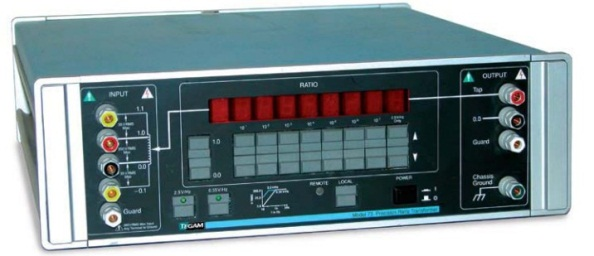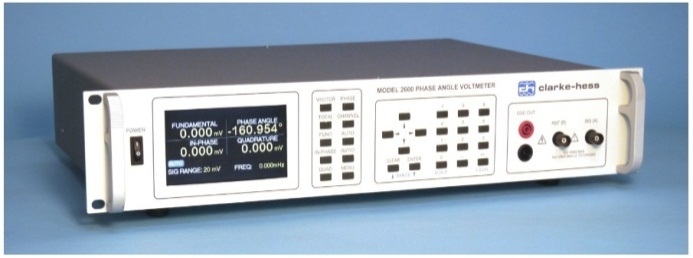News/Events

# RESOLVERS TESTING SOLUTION

## Synchro-resolvers: These are electromechanical angle to ratio devices used in many aircraft control systems. Because of this important application, the operation with high accuracy is needed to test usually.

The chart below is the testing configuration include: Conference, resolvers, Ratio Transformer (Tegam model PRT-73), Phase angle meter (Clarke-Hess model 2600)• S4 of the resolver is connected to the input of the ratio transformer
• S3 and S2 are connected to the common input of the ratio transformer
• S1 is connected to one of the (PAV) signal input terminals

Testing Procedure

• The shaft is precisely set to a known angle. The ratio transformer is set to the tangent of the shaft angle
• S4 is multiplied by the ratio setting of the ratio transformer. Since S4 is the cosine output of the resolver, the output of the ratio transformer should equal the sine output.
• S1 is the sine output of the resolver; the output of the ratio should equal the S1 output of the resolver.
• If two outputs are equal, the PAV (Phase Angle Voltmeter) will indicate a null condition.
• If the PAV does not indicate a null condition, the setting of the ratio transformer is adjusted until a null condition is indicated.
• The arctangent of the ratio transformer setting is the angle that the resolver output is indicating. Comparing this angle with the set angle of resolver shaft to find the error of the resolver.

The setup above only works for angles 0 ~ 45˚ and 180 ~ 225˚. Other angles can still be tested by changing the resolver output connections as the table below:

NM:  Input of Null Meter

C: Input Common of the ratio transformer

RT: Input of the ratio transformer

 Angle S1 S3 S4 S2 0 to 45 NM C RT C 45 to 90 RT C NM C 90 to 135 RT C C NM 135 to 180 NM C C RT 180 to 225 NM C RT C 225 to 270 RT C NM C 270 to 315 RT C C NM 315 to 360 NM C C RT

Main Features and specifications of instruments in the system

Ratio Transformer: PRT – 73 (Tegam)• Remotely programmable via standard IEEE-488 interface , support for automatic testing procedure
• Storing testing procedure for the repeatability of measurements.
• Range of ratio setting: -0,0010000 ~ 1,0009999
• Standard resolution: 0,1ppm(0,0000057˚), Optional: 0,01ppm
• Wide bandwidth: 50Hz ~ 20kHz
• Maximum input voltage: 350V (0,35V/Hz)

Phase Angle Voltmeter: 2600 (Clarke-Hess)• Channels: 2
• Mode of measurements: Total, Fundamental, In-phase, Quadrature, Ratio, Frequency and Phase
• Voltage input Range: 20mV rms ~ 630V rms
• Phase Input Ranges: 0˚ ~ 360˚ or ±180˚
• Accuracy: 0,01˚ (pha)
• Resolution: 4 ½ digit (Voltage), 6 digit (Phase)
• Frequency Range: 20Hz ~ 100kHzN

Source: TMC